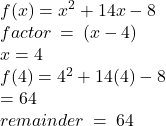## What is the remainder when f(x) = x2 + 14x − 8 is divided by (x − 4)? 80 64 56 52

Question

What is the remainder when f(x) = x2 + 14x − 8 is divided by (x − 4)?

80
64
56
52

in progress 0
5 months 2021-08-31T05:42:03+00:00 2 Answers 3 views 0